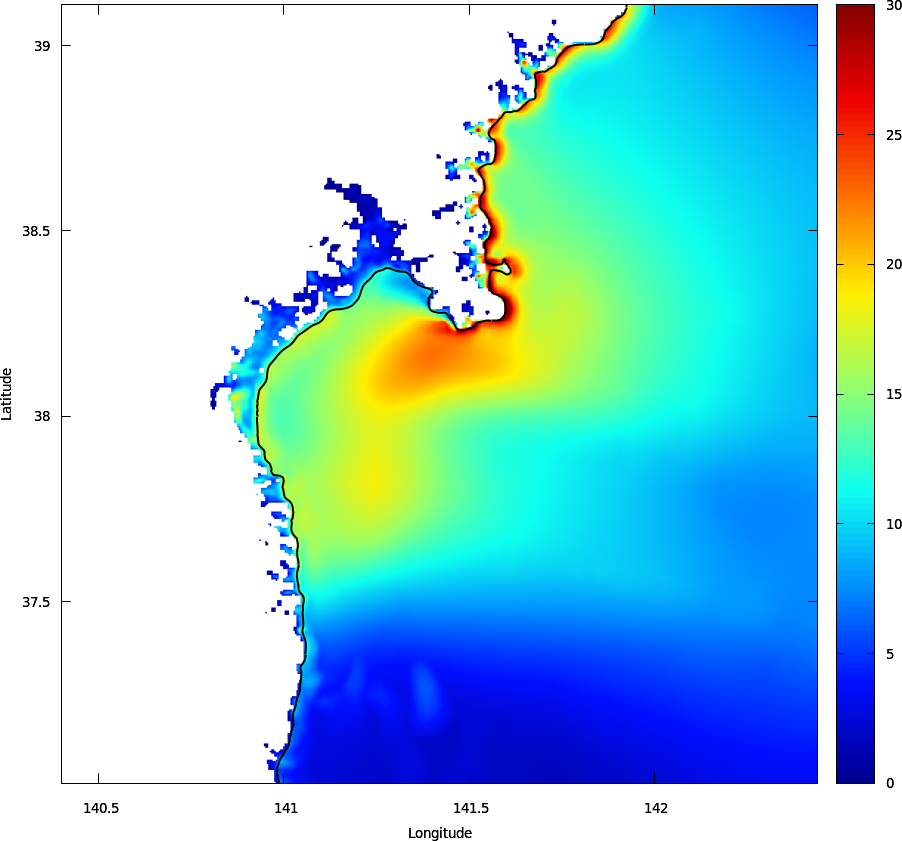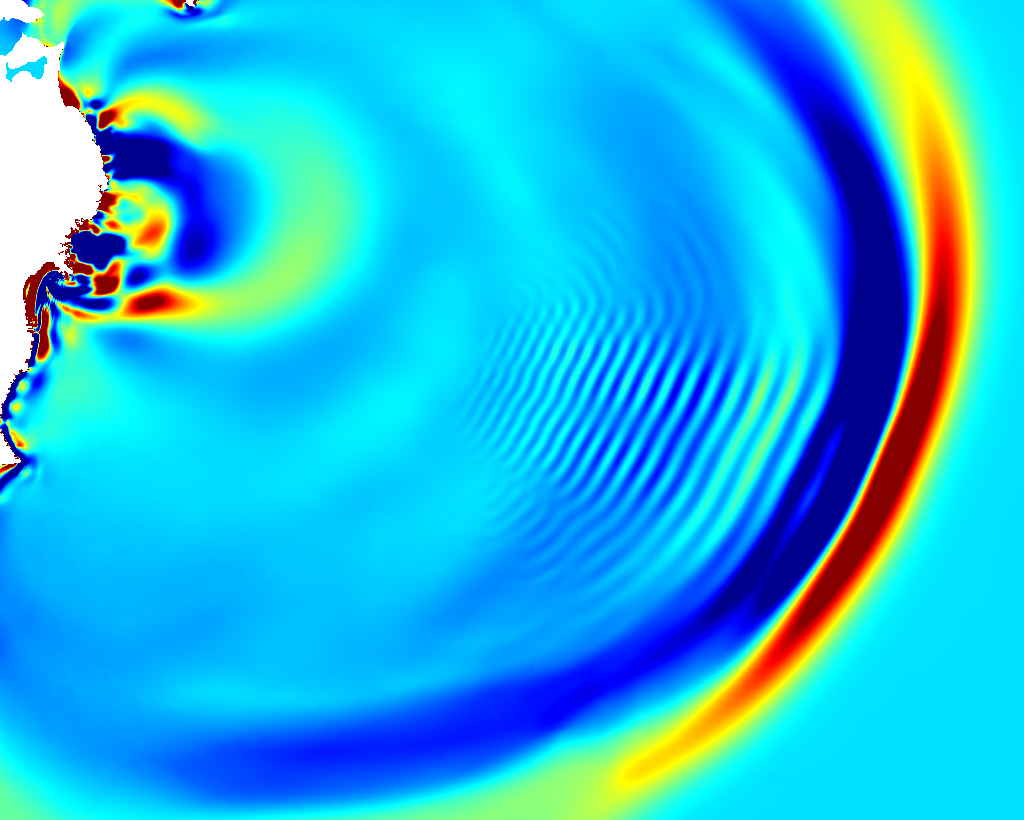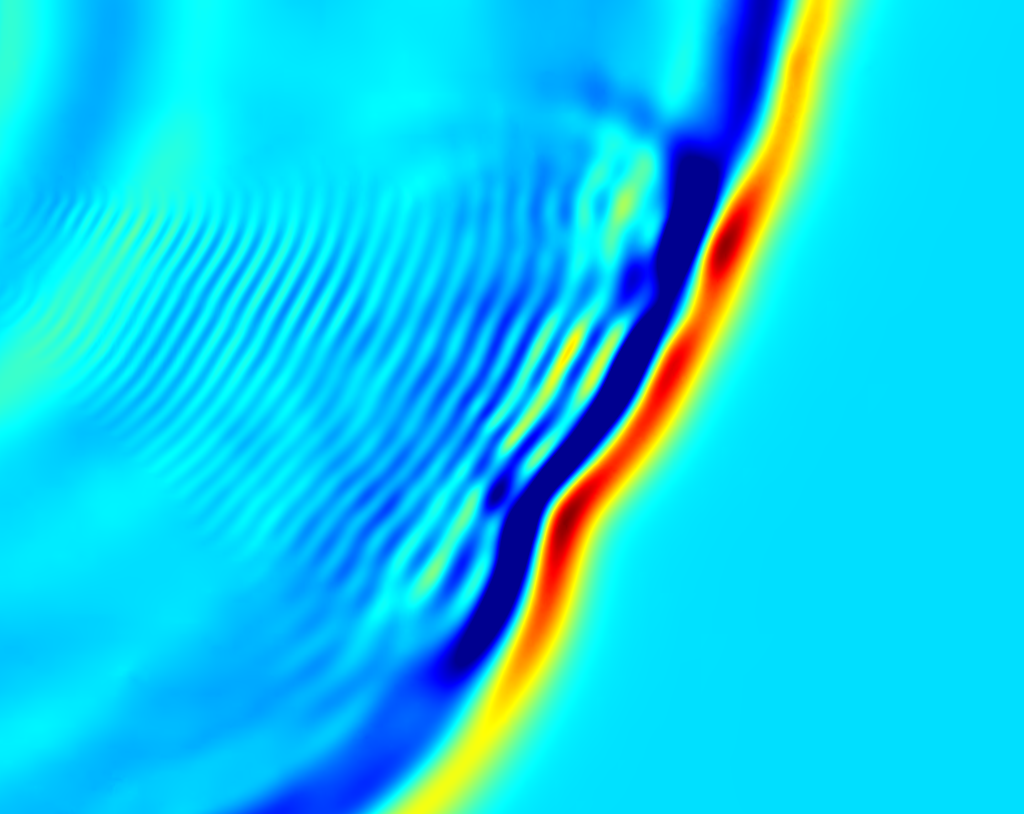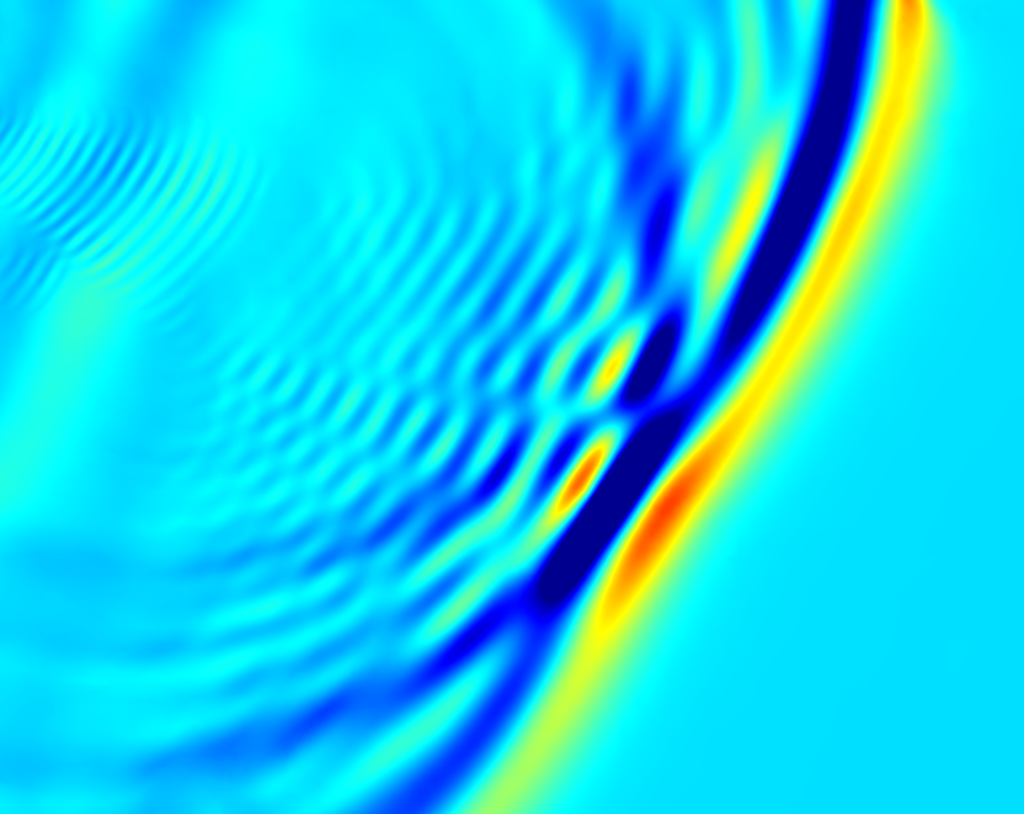# The 2011 Tohoku tsunami

The 11th March 2011 Tohoku tsunami was triggered by a fault rupture off the east coast of Japan. It is modelled here using a setup similar to that used for the 2004 tsunami, where more comments can be found.

A significant difference is that we use a much more realistic fault model obtained through seismic inversion of the actual earthquake.

See Popinet, 2012 for a detailed discussion of the pure Saint-Venant model, Popinet, 2015 for a discussion of the dispersive effects modelled using the Green–Naghdi equations and Popinet, 2019 for the application of the single-layer dispersive model.

## Results

 Your browser does not support the video tag. Animation of the wave elevation. Dark blue is -1 metre and less. Dark red is +2 metres and more. The domain is approximately 6000 \times 8000 km. Your browser does not support the video tag. Animation of the level of refinement. Dark blue is 5 (~250 km) and dark red is 13 (~1 km).
 Your browser does not support the video tag. Animation of the wave elevation. Detail for the Sendai region. The area shown is approximately 140 \times 100 km. Dark blue is -5 metres and less. Dark red is +5 metres and more. Your browser does not support the video tag. Detail for the Fukushima region. Your browser does not support the video tag. Detail for the Miyako region. Your browser does not support the video tag. Detail for the Ofunato region.
set term pngcairo enhanced size 1024,1024 font ",10"
set output 'flooding.png'
set pm3d map
set palette defined ( 0 0 0 0.5647, 0.125 0 0.05882 1, 0.25 0 0.5647 1, \
0.375 0.05882 1 0.9333, 0.5 0.5647 1 0.4392, \
0.625 1 0.9333 0, 0.75 1 0.4392 0, \
0.875 0.9333 0 0, 1 0.498 0 0 )
set multiplot
unset key
set size ratio -1
set xrange [140.4:142.44]
set yrange [37.01:39.11]
set xlabel 'Longitude'
set ylabel 'Latitude'
set cbrange [0:30]
splot 'flooding' u 1:2:($3 > 1e-3 ? ($4 < 0. ? $5 :$5 - $4) : 1e1000) set contour base set cntrparam levels discrete 0 set cntrlabel onecolor unset surface splot 'flooding' u 1:2:4 lt 3 lc rgb "#000000" lw 2 unset multiplot ! mogrify -trim +repage flooding.pngFlooding in the Sendai, Ofunato and Fukushima districts (script) The following three figures highlight the importance of dispersive effects after one, two and three hours (see Popinet, 2015 for a discussion).Leading wave front after three hours ## Solver setup See tsunami.c for more detailed explanations. If GN is defined we use the Green–Naghdi solver, otherwise the multilayer solver (hydrostatic if HYDRO is defined). To run the three cases use: make tohoku.tst tohoku-gn.tst tohoku-hydro.tst #include "spherical.h" #if GN # include "green-naghdi.h" # define MGD mgD.i #else # include "layered/hydro.h" # if HYDRO # define MGD 0 # else # include "layered/nh.h" # define MGD mgp.i # endif # include "layered/filter.h" # include "layered/perfs.h" scalar h; vector u; #endif #include "terrain.h" #include "okada.h" #define MAXLEVEL 13 #define MINLEVEL 5 #define ETAE 1e-2 // error on free surface elevation (1 cm) #define ETAMAXE 5e-2 // error on maximum free surface elevation (5 cm) int main() { // Earth radius in metres Radius = 6371220.; // the domain is 73 degrees squared size (73.); // centered on 142,38 longitude,latitude origin (142. - L0/2., 38. - L0/2.); // acceleration of gravity in m/min^2 G = 9.81*sq(60.); // 32^2 grid points to start with init_grid (1 << MINLEVEL); For the Green-Naghdi solver we do only one iteration of the multigrid solver to speed things up. This does not change the solution. For the multilayer solver we use a grid-scale filter with a 3 minutes timescale. This is not strictly necessary but makes nicer pictures (it damps grid-scale noise). In the non-hydrostatic case, we add a cut-off breaking parameter (Popinet, 2019). #if GN TOLERANCE = HUGE; NITERMIN = 1; #else // !GN filter = 3.; // 3 minutes filter #if NH breaking = 0.07; #endif #endif // !GN run(); } scalar etamax[]; int my_adapt() { #if QUADTREE scalar eta[]; foreach() eta[] = h[] > dry ? h[] + zb[] : 0; boundary ({eta}); astats s = adapt_wavelet ({eta, etamax}, (double[]){ETAE,ETAMAXE}, MAXLEVEL, MINLEVEL); fprintf (ferr, "# refined %d cells, coarsened %d cells\n", s.nf, s.nc); return s.nf; #else // Cartesian return 0; #endif } ## Terrain databases We use both the ETOPO2 topography and SRTM land data for Japan. See the xyz2kdt manual to generate the ETOPO2 database. The SRTM database can be generated using the following script (which will need to be adapted as the USGS often changes its website) # SRTM_resultExport.csv from http://edcsns17.cr.usgs.gov/NewEarthExplorer/ # see also http://gfs.sourceforge.net/wiki/index.php/Xyz2kdt files=awk 'BEGIN{FS="\"|,"}{ if ($2!="ENTITY_ID") print $2;}' < SRTM_resultExport.csv | \ sed 's/SRTM3//g' | \ awk '{ print "http://dds.cr.usgs.gov/srtm/version2_1/SRTM3/Eurasia/"$1 ".hgt.zip" }'
for f in $files; do wget$f
done

cc pkg-config glib-2.0 --cflags --libs -Wall -O2 srtm2kdt.c -o srtm2kdt
for f in *.zip; do
p=echo $f | sed 's/.*$$[NS]$$$$[0-9][0-9]$$$$[WE]$$$$[0-9][0-9][0-9]$$\.hgt\.zip/\1 \2 \3 \4/' lat=echo$p | awk '{if ($1 == "S") print -$2; else print $2;}' lon=echo$p | awk '{if ($3 == "W") print -$4; else print $4;}' echo$f >> /dev/stderr
unzip -c -q $f | ./srtm2kdt$lon $lat done | xyz2kdt -v$HOME/terrain/srtm_japan

with the following strm2kdt.c code:

#include <stdio.h>
#include <assert.h>
#include <stdlib.h>
#include <glib.h>

#define NCOLS 1201
#define NROWS 1201
#define CELLSIZE (1./(NCOLS - 1))
#define NODATA_VALUE -32768

int main (int argc, char * argv[])
{
double lat, lon;
gint16 v, vs;
int i, j;

double xllcorner = atof (argv);
double yllcorner = atof (argv);

for (j = 0; j < NROWS; j++) {
lat = yllcorner + CELLSIZE*(NROWS - 1 - j);
for (i = 0; i < NCOLS; i++) {
lon = xllcorner + CELLSIZE*i;
assert (fread (&v, sizeof (gint16), 1, stdin));
vs = GINT16_FROM_BE (v);
if (vs > 0)
printf ("%.8f %.8f %d\n", lon, lat, vs);
}
//    fprintf (stderr, "\rRow %d/%d              ", j + 1, NROWS);
}
//  fputc ('\n', stderr);
return 0;
}

## Initial conditions

event init (i = 0)
{
#if !GN
h = hl;
u = ul;
#endif

terrain (zb, "~/terrain/etopo2", "~/terrain/srtm_japan", NULL);

if (restore (file = "restart"))
conserve_elevation();
else {
conserve_elevation();

foreach()
h[] = max(0., - zb[]);
boundary ({h});

The initial deformation is given by a long collection of small Okada subfaults, obtained from seismic inversion (Popinet, 2012).

    #include "tohoku/faults.h"
}

}

event logfile (i++) {
stats s = statsf (h);
norm n = normf (u.x);
if (i == 0)
fprintf (ferr,
"t i h.min h.max h.sum u.x.rms u.x.max dt mgD.i speed tn\n");
fprintf (ferr, "%g %d %g %g %g %g %g %g %d %g %ld\n",
t, i, s.min, s.max, s.sum, n.rms, n.max, dt, MGD,
perf.speed, grid->tn);
}

event viscous_term (i++)
{
foreach() {
double a = h[] < dry ? HUGE : 1. + 1e-4*dt*norm(u)/h[];
foreach_dimension()
u.x[] /= a;
if (h[] > dry && h[] + zb[] > etamax[])
etamax[] = h[] + zb[];
}
boundary ({etamax, u});
}

## Outputs

event snapshots (t += 15) {
char name;
sprintf (name, "dump-%g", t);
dump (name);
}

event flooding (t = 390)
{
FILE * fp = fopen ("flooding", "w");
output_field ({h,zb,etamax}, fp,
box = {{140.4,37.01},{142.44,39.11}},
n = 512, linear = true);
}

event figures (t = 60; t <= 180; t += 60)
{
scalar m[], etam[];
foreach() {
etam[] = eta[]*(h[] > dry);
m[] = etam[] - zb[];
}
boundary ({m, etam});
char name;
sprintf (name, "eta-%g.png", t);
output_ppm (etam, mask = m, min = -1, max = 2, file = name, n = 1024,
linear = true, box = {{123,14},{177,55}},
opt = "-fill white -opaque black");

sprintf (name, "level-%g.png", t);
scalar l[];
foreach()
l[] = level;
output_ppm (l, min = 5, max = 13, file = name, n = 1024,
linear = false, box = {{123,14},{177,55}});

if (t == 60)
output_ppm (etam, mask = m, min = -1, max = 2, file = "zoom-60.png",
n = 1024,
linear = true, box = {{140.6,30.8},{154.6,42}},
opt = "-fill white -opaque black");
else if (t == 120)
output_ppm (etam, mask = m, min = -1, max = 2, file = "zoom-120.png",
n = 1024,
linear = true, box = {{151.8,27.56},{165.8,38.68}},
opt = "-fill white -opaque black");
else if (t == 180)
output_ppm (etam, mask = m, min = -1, max = 2, file = "zoom-180.png",
n = 1024,
linear = true, box = {{158.8,24.25},{172.7,35.3}},
opt = "-fill white -opaque black");
}

event movies (t += 0.5)
{
scalar m[], etam[];
foreach() {
etam[] = eta[]*(h[] > dry);
m[] = etam[] - zb[];
}
boundary ({m, etam});
output_ppm (etam, mask = m, min = -1, max = 2,
n = 1024, linear = true, file = "eta.mp4");

output_ppm (etam, mask = m, min = -5, max = 5,
n = 1024, linear = true,
box = {{140.4,37.51},{142.44,38.61}},
file = "eta-sendai.mp4");

output_ppm (etam, mask = m, min = -5, max = 5,
n = 1024, linear = true,
box = {{140.5,36.53},{142.52,37.62}},
file = "eta-fukushima.mp4");

output_ppm (etam, mask = m, min = -5, max = 5,
n = 1024, linear = true,
box = {{141.32,39.42},{143.44,40.50}},
file = "eta-miyako.mp4");

output_ppm (etam, mask = m, min = -5, max = 5,
n = 1024, linear = true,
box = {{141.11,38.51},{143.19,39.60}},
file = "eta-ofunato.mp4");

scalar l = etam;
foreach()
l[] = level;
output_ppm (l, min = MINLEVEL, max = MAXLEVEL, n = 1024,
file = "level.mp4");

#if _OPENMP
foreach()
etam[] = pid();
double tmax = omp_get_max_threads() - 1;
output_ppm (etam, max = tmax, n = 512, file = "pid.mp4");
#endif // _OPENMP
}

This file includes the locations of various wave gauges.

#include "tohoku/gauges.h"

event adapt (i++) my_adapt();